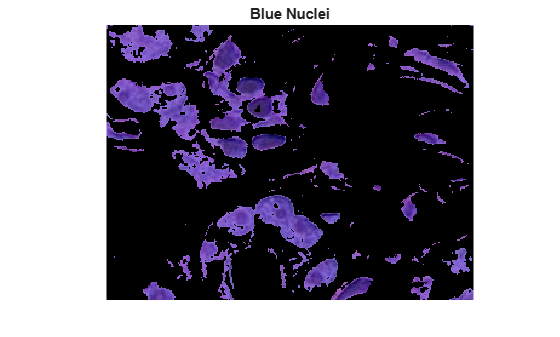# 使用 K 均值聚类实现基于颜色的分割

### 步骤 1：读取图像

`hestain.png` 中读取，这是一个带有苏木精和曙红染色组织 (H&E) 的图像。这种染色方法有助于病理学家区分染成蓝-紫色和粉红色的组织类型。

```he = imread("hestain.png"); imshow(he) title("H&E Image") text(size(he,2),size(he,1)+15, ... "Image courtesy of Alan Partin, Johns Hopkins University", ... FontSize=7,HorizontalAlignment="right")```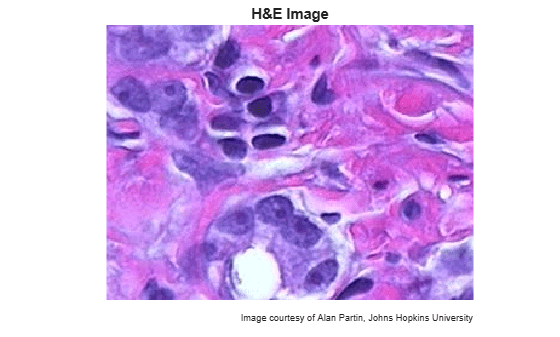### 步骤 2：用 K 均值聚类对 RGB 颜色空间的颜色进行分类

```numColors = 3; L = imsegkmeans(he,numColors); B = labeloverlay(he,L); imshow(B) title("Labeled Image RGB")```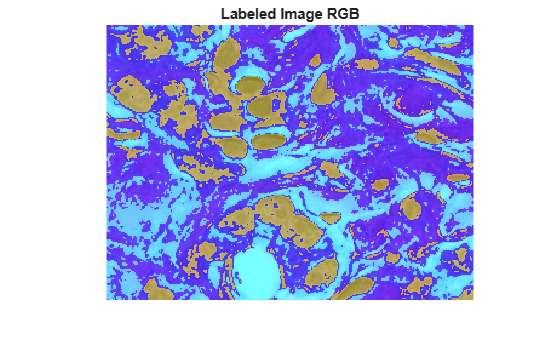### 步骤 3：将图像从 RGB 颜色空间转换为 L*a*b* 颜色空间

L*a*b* 颜色空间将图像的光度和颜色分开。这使得按颜色分割区域变得更加容易并且与亮度无关。颜色空间也更符合人类对图像中不同的白色、蓝-紫色和粉色区域的视觉感知。

L*a*b* 颜色空间是从 CIE XYZ 三色值派生的。L*a*b* 空间包含光度层 L*、色度层 a*（表示颜色落在沿红-绿轴的位置）和色度层 b*（表示颜色落在沿蓝-黄轴的位置）。所有颜色信息都在 a* 和 b* 层。

`lab_he = rgb2lab(he);`

### 步骤 4：用 K 均值聚类对基于 a*b* 空间的颜色进行分类

```ab = lab_he(:,:,2:3); ab = im2single(ab); pixel_labels = imsegkmeans(ab,numColors,NumAttempts=3);```

```B2 = labeloverlay(he,pixel_labels); imshow(B2) title("Labeled Image a*b*")```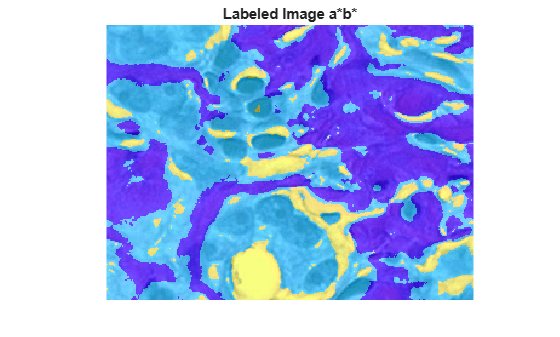### 步骤 5：创建按颜色分割 H&E 图像的图像

```mask1 = pixel_labels == 1; cluster1 = he.*uint8(mask1); imshow(cluster1) title("Objects in Cluster 1");```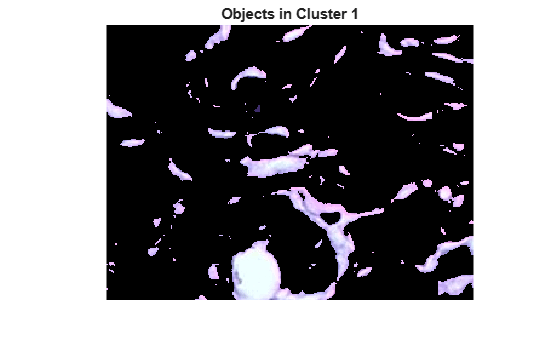```mask2 = pixel_labels == 2; cluster2 = he.*uint8(mask2); imshow(cluster2) title("Objects in Cluster 2");```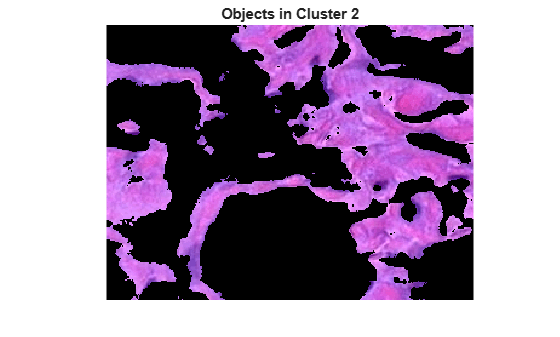```mask3 = pixel_labels == 3; cluster3 = he.*uint8(mask3); imshow(cluster3) title("Objects in Cluster 3");```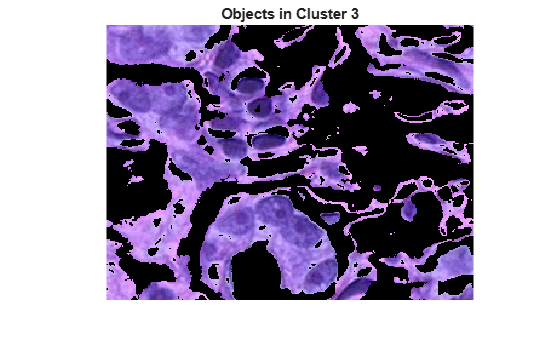### 步骤 6：分割核

L* 层包含每个像素的亮度值。提取此簇中像素的亮度值，并使用 `imbinarize` 函数用全局阈值对其设置阈值。掩膜 `idx_light_blue` 给出了浅蓝色像素的索引。

```L = lab_he(:,:,1); L_blue = L.*double(mask3); L_blue = rescale(L_blue); idx_light_blue = imbinarize(nonzeros(L_blue));```

```blue_idx = find(mask3); mask_dark_blue = mask3; mask_dark_blue(blue_idx(idx_light_blue)) = 0; blue_nuclei = he.*uint8(mask_dark_blue); imshow(blue_nuclei) title("Blue Nuclei")```